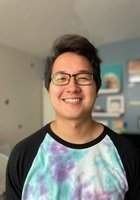1 of 7

Connect with hundreds of tutors like Casey

Expert tutoring for students at all levels

150,000+ clients. 4.9 / 5.0 Rating of Tutoring Sessions

Featured by
Tutors fromCasey

Certified Tutor

I am a graduate student in Applied Mathematics with 4+ years experience tutoring and hosting recitations. Classes that I have tutored in the past include algebra, trigonometry, calculus (I-III), differential equations, and linear algebra. I have 6 years of MATLAB experience, and have taught a recitation in programming in MATALB as well.

Casey’s Qualifications

Education & Certification

Undergraduate Degree: Arizona State University - Bachelor of Science, Mathematics

Graduate Degree: Arizona State University - Doctor of Philosophy, Applied Mathematics

Test Scores

GRE Quantitative: 167

Hobbies

Video games, music, going on walks.

Tutoring Subjects

Algebra

Algebra 2

AP Calculus AB

AP Calculus BC

Calculus

Calculus 2

Calculus 3

Differential Equations

Discrete Math

Linear Algebra

Math

MATLAB

Multivariable Calculus

Numerical Analysis

Productivity

Real Analysis

Technology and Coding

Trigonometry